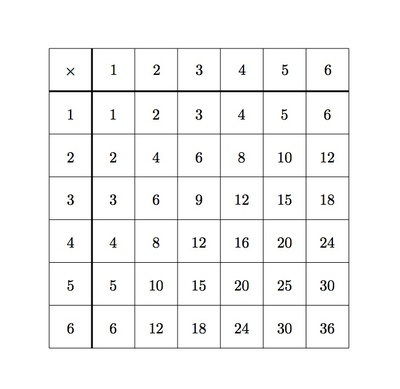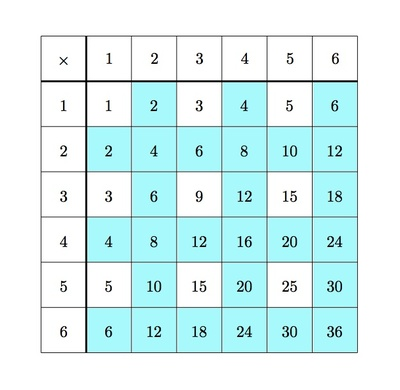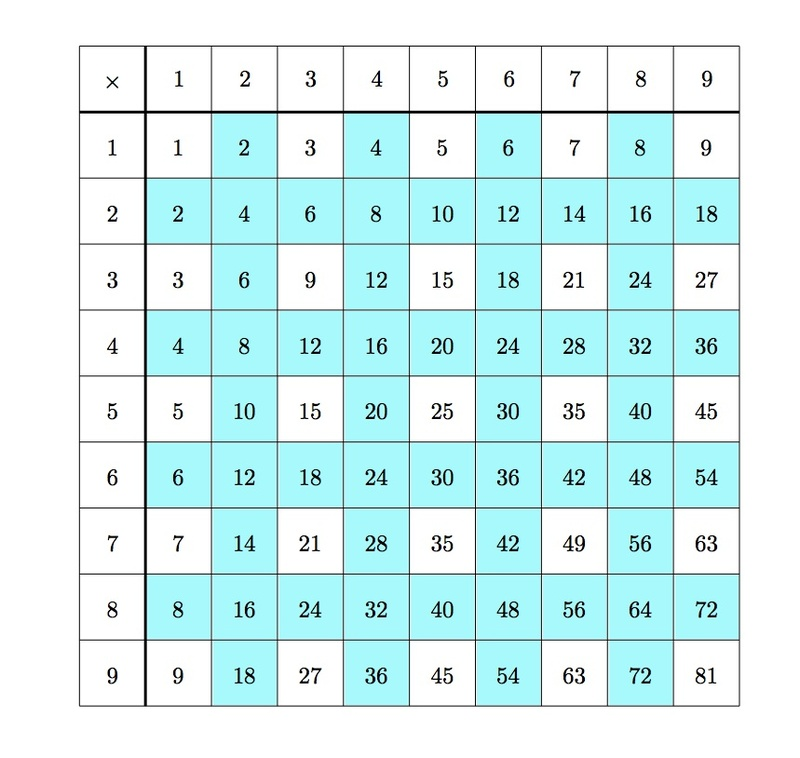# Patterns in the multiplication table

Alignments to Content Standards: 3.OA.D.9

The table shows products of the whole numbers 1 through 6.1. Color all of the even products in the table.
2. Sometimes there are even numbers next to each other in the table. However, there are never odd numbers next to each other. Why is this true?

3. Imagine the same kind of table that shows all the ways of multiplying two numbers between 1 and 9. Would this still be true?

## IM Commentary

This task is intended for instruction. The goal is to look for structure and identify patterns and then try to find the mathematical explanation for this. This problem examines the ''checkerboard'' pattern of even and odd numbers in a single digit multiplication table. The even numbers in the table are examined in depth using a grade appropriate notion of even, namely the possibility of reaching the number counting by $2$'s or expressing the number as a whole number of pairs.

The multiplication table itself is a little hard to parse because it has so much information in it. The teacher should point out that the first row and first column are not part of the table itself but show what numbers are being multiplied. In general, the students will probably need a lot of help understanding what the task is asking. Students are most likely to benefit if they can work with a partner or in small groups on this task.

Although not requested in the problem, teachers may wish to investigate further why a product of an odd number by another odd number appears to always be odd. We can see why by looking closely at $5 \times 3$ as an example. We can view this, using the distributive property, as

\begin{align} (4+1) \times (2 + 1) = 4 \times 2 + 1 \times 2 + 4 \times 1 + 1 \times 1 \end{align}

Now $4 \times 2, 1 \times 2, 4 \times 1$ are all even numbers because they appear in an even row or an even column of the table. A sum of even numbers is even because a whole number of pairs added to another whole number of pairs is a whole number of pairs. So $5 \times 3$ can be expressed as an even number plus one. This is an odd number because there is one left over when all possible pairs have been removed. Any conversation in this direction should be accompanied by pictures since students are not ready for such abstract arguments using only words and symbols.A more sophisticated version of this task is found in "4.OA Identifying Multiples" where the same pattern is considered not only for even and odd numbers but also for multiples of $3$ and $4$.

## Solution

1. Below the even numbers in the table have been shaded blue:2. For smaller numbers such as $2,4,6,8$ students will know from experience that these numbers are even. For a larger number such as $24 = 4 \times 6$, a good way to visualize why this is an even number would be to view the number $6$ as made up of $3$ pairs: then multiplying by $4$ means that we take $4$ groups of $6$:and so $4$ groups of $3$ pairs. This is an even number since it is $4 \times 3$ pairs.

Similar reasoning will work in other cases. For a product such as $6 \times 5$, we could first use the commutative property of multiplication to give $6 \times 5 = 5 \times 6$ and then use the same reasoning we used to see why $4 \times 6$ is even.

If we look, for example, at the second column, this contains the numbers $1 \times 2, 2 \times 2, \ldots, 6 \times 2$: these are all even numbers because we can visualize these as one pair, two pair, $\ldots$, six pairs. Similarly, in the $4^{\rm th}$ row we have $4 \times 1, 4 \times 2, \ldots, 4 \times 6$. Using the commutative property of multiplication, this is the same as $1 \times 4, 2 \times 4, \ldots, 6 \times 4$. Since $4$ can be divided evenly into two pair, all of these numbers can be divided evenly into pairs and so they are all even. So even numbered rows and columns contain only even numbers.

We know from above that even numbered rows and even numbered columns contain only even numbers. We also know that every other column/row is even numbered because the even numbers are found counting by $2$'s which skips one whole number each time. Adjacent columns cannot contain odd numbers because one of the columns is an even column (which contains only even numbers) and one is an odd column. For the same reason, adjacent rows cannot contain odd numbers.

3. The reasoning in part (b) applies no matter how far the multiplication table is extended. This is the case because the reasoning depends only on whether the numbers considered are even or odd. The $9$ by $9$ table is pictured below: